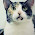## 1/23/2013

### Floating point vs. integer arithmetics

Eventually, the chess engine will be run on the GPU. The CUDA-C code (assuming that is the language of choice) has been compiled into machine instructions. Some instructions will take longer time than others. While full utilization of parallel threads and planing memory accesses carefully are most important things, the instructions used also count when optimizing the performance. One important thing to notice about the GPU is that the same operations with different data types take different amount of time.

GPUs have been designed for fast floating point arithmetics. Unfortunately, chess engines do integer arithmetics and bit twiddling. It should be clear that the nominal top speeds (in floating-point operations per second or FLOPS) given for the GPUs in the technical specifications cannot be achieved with integer operations. Even all the floating point operations will not do that. There are some special instructions like multiply-add that perform two operations at once to produce the supercomputer-like teraflops figures in the data sheets.

So let us compare integer operations with 32-bit and 64-bit floating point operations (assume CUDA compute capability 2.0). The table shows clock cycles per thread taken by the instruction

Operation32-bit float64-bit float32-bit integer
Multiply122
Logical op.N/AN/A1
ShiftN/AN/A2
Type conv.111

The situation is not as bad as it could be. Additions and logical operations are fast. Multiplications and shifts are only twice as slow. Multiply-add is useful in the address calculations and it is executed with the same cost as a simple multiply.

The situation is not optimal for the bitboard representation often used by chess engines. The all-important shift operations cost twice the other operations, and there is no native 64-bit integer. Other board representations may be more efficient, but it is hard to say without testing it out.

One thing that should be avoided is unnecessary casting between data types. Although a cast operation takes only one clock cycle per thread, these additional instructions pile up and slow down the execution. The compiler might be stupid in the sense that it sometimes adds cast instructions when they are not really necessary. This can be seen by examining the PTX-assembler code produced by the compiler. It might be required that the inner loops of the search algorithm are written directly in assembler to avoid this waste of instructions.

As a conclusion, it can be said that the speed of integer arithmetics is not going to be an issue.

1.2.